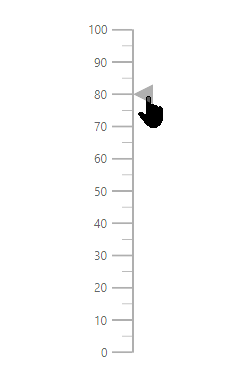Search results

# User Interaction in ASP.NET Core Linear Gauge control

20 Oct 2021 / 3 minutes to read

## Tooltip

Linear Gauge displays the details about a pointer value through `e-lineargauge-tooltip`, when the mouse hovers over the pointer. To enable the tooltip, set `Enable` property as true.

tagHelper
``````@using Syncfusion.EJ2.LinearGauge

<ejs-lineargauge id="gauge">
<e-lineargauge-tooltip Enable="true"></e-lineargauge-tooltip>
<e-lineargauge-axes>
<e-lineargauge-axis>
<e-lineargauge-pointers>
<e-lineargauge-pointer Value="80"></e-lineargauge-pointer>
</e-lineargauge-pointers>
</e-lineargauge-axis>
</e-lineargauge-axes>
</ejs-lineargauge>``````

<<<<<<< HEAD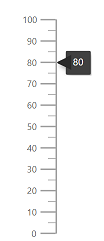=======25c536e4a77a22da0ab7e1d0d5fc4cc724190ae6

### Tooltip format

Tooltip in the Linear Gauge control can be formatted using the `Format` property in `e-lineargauge-tooltip`. It is used to render the tooltip in certain format or to add a user-defined unit in the tooltip. By default, the tooltip shows the pointer value only. In addition to that, more information can be added in the tooltip. For example, the format {value}km shows pointer value with kilometer unit in the tooltip.

tagHelper
``````using Syncfusion.EJ2.LinearGauge

<ejs-lineargauge id="gauge">
<e-lineargauge-tooltip Enable="true" Format="{value}km"></e-lineargauge-tooltip>
<e-lineargauge-axes>
<e-lineargauge-axis>
<e-lineargauge-pointers>
<e-lineargauge-pointer Value="80"></e-lineargauge-pointer>
</e-lineargauge-pointers>
</e-lineargauge-axis>
</e-lineargauge-axes>
</ejs-lineargauge>``````

<<<<<<< HEAD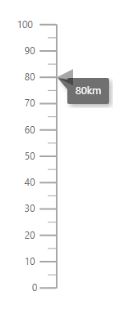=======25c536e4a77a22da0ab7e1d0d5fc4cc724190ae6

### Tooltip Template

The HTML element can be rendered in the tooltip of the Linear Gauge using the `Template` property in `e-lineargauge-tooltip`. The \${value} can be used as placeholders in the HTML element to display the pointer values of the corresponding axis.

tagHelper
``````@using Syncfusion.EJ2.LinearGauge

<ejs-lineargauge id="gauge">
<e-lineargauge-tooltip Enable="true" Template="<div>Pointer: 80 </div>"></e-lineargauge-tooltip>
<e-lineargauge-axes>
<e-lineargauge-axis>
<e-lineargauge-pointers>
<e-lineargauge-pointer Value="80"></e-lineargauge-pointer>
</e-lineargauge-pointers>
</e-lineargauge-axis>
</e-lineargauge-axes>
</ejs-lineargauge>``````

<<<<<<< HEAD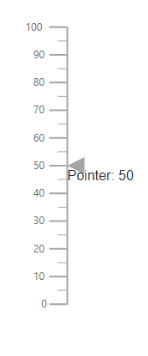=======25c536e4a77a22da0ab7e1d0d5fc4cc724190ae6

### Customize the appearance of the tooltip

The tooltip can be customized using the following properties in `e-lineargauge-tooltip`.

tagHelper
``````@using Syncfusion.EJ2.LinearGauge

<ejs-lineargauge id="gauge">
<e-lineargauge-tooltip Enable="true" Fill="#e5bcbc">
<e-tooltipsettings-border Width="2" Color="#d80000"></e-tooltipsettings-border>
</e-lineargauge-tooltip>
<e-lineargauge-axes>
<e-lineargauge-axis>
<e-lineargauge-pointers>
<e-lineargauge-pointer Value="80"></e-lineargauge-pointer>
</e-lineargauge-pointers>
</e-lineargauge-axis>
</e-lineargauge-axes>
</ejs-lineargauge>``````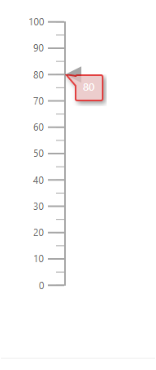## Positioning the tooltip

The tooltip is positioned at the End of the pointer. To change the position of the tooltip at the start, or center of the pointer, set the `Position` property to Start or Center.

tagHelper
``````@using Syncfusion.EJ2.LinearGauge

<ejs-lineargauge id="gauge">
<e-lineargauge-tooltip Enable="true" Position="Center">
</e-lineargauge-tooltip>
<e-lineargauge-axes>
<e-lineargauge-axis>
<e-lineargauge-pointers>
<e-lineargauge-pointer Value="50" Type="Bar" Color="blue"></e-lineargauge-pointer>
</e-lineargauge-pointers>
</e-lineargauge-axis>
</e-lineargauge-axes>
</ejs-lineargauge>``````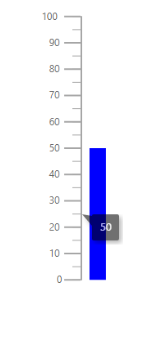## Pointer Drag

To drag either marker or bar pointer to the desired axis value, set the `EnableDrag` property as true in `e-lineargauge-pointer`.

tagHelper
``````@using Syncfusion.EJ2.LinearGauge

<ejs-lineargauge id="gauge">
<e-lineargauge-axes>
<e-lineargauge-axis>
<e-lineargauge-pointers>
<e-lineargauge-pointer EnableDrag="true" Value="80"></e-lineargauge-pointer>
</e-lineargauge-pointers>
</e-lineargauge-axis>
</e-lineargauge-axes>
</ejs-lineargauge>``````